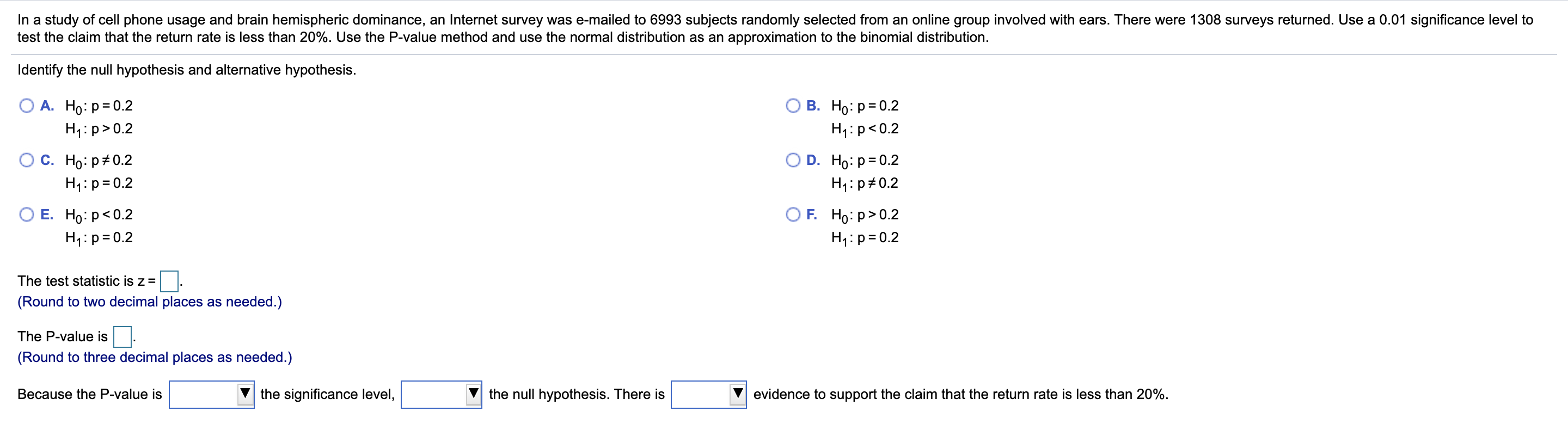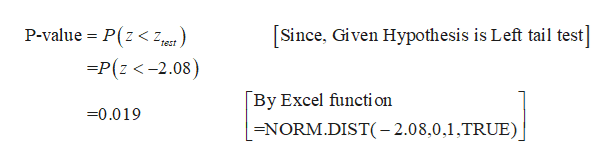# In a study of cell phone usage and brain hemispheric dominance, an Internet survey was e-mailed to 6993 subjects randomly selected from an online group involved with ears. There were 1308 surveys returned. Use a 0.01 significance level totest the claim that the return rate is less than 20%. Use the P-value method and use the normal distribution as an approximation to the binomial distribution.Identify the null hypothesis and alternative hypothesis.В. Но: р3D0.2А. Но: р3D0.2H1:p>0.2H1:p 0.2Е. Но: р

Question
4 viewshelp_outlineImage TranscriptioncloseIn a study of cell phone usage and brain hemispheric dominance, an Internet survey was e-mailed to 6993 subjects randomly selected from an online group involved with ears. There were 1308 surveys returned. Use a 0.01 significance level to test the claim that the return rate is less than 20%. Use the P-value method and use the normal distribution as an approximation to the binomial distribution. Identify the null hypothesis and alternative hypothesis. В. Но: р3D0.2 А. Но: р3D0.2 H1:p>0.2 H1:p<0.2 С. Но: р#0.2 O D. Ho: p= 0.2 H4:p=0.2 H1: p#0.2 O F. Но: р> 0.2 Е. Но: р<0.2 H4:p=0.2 H4:p=0.2 The test statistic is z = (Round to two decimal places as needed.) The P-value is (Round to three decimal places as needed.) Because the P-value is the significance level, the null hypothesis. There is evidence to support the claim that the return rate is less than 20%. fullscreen
check_circle

Step 1

From the given information, the claim of the problem is the return rate is less than 20%.

Null hypothesis:

H0p=0.2.

That is, there is no significance evidence that the return rate is less than 20%.

Alternative hypothesis:

H1p<0.2.

That is, there is a significance evidence that the return rate is less than 20%.

The correct option is B. H0p=0.2 and H1p<0.2.

From the given information, in a sample of 6993 respondents, 1308 of them are returned.

The sample size is, n =6993 and x=1308. The sample proportion is   =0.19(=1308/6993)

The test statistic is  ̶ 2.08 and it is calculated below

Step 2

The P-value is 0.019 and it is calcula...help_outlineImage TranscriptioncloseP-value = P(z < Z,est ) [Since, Given Hypothesis is Left tail test] =P(z <-2.08) [By Excel functi on =0.019 =NORM.DIST(- 2.08,0,1,TRUE) fullscreen

### Want to see the full answer?

See Solution

#### Want to see this answer and more?

Solutions are written by subject experts who are available 24/7. Questions are typically answered within 1 hour.*

See Solution
*Response times may vary by subject and question.
Tagged in

### Hypothesis Testing